# 蒙特卡罗方法在强化学习中的应用

### 前文的简要总结及算法改进方法

• 易于开发交易算法和高“学习”速度。强化学习（RL）可以很容易地融入到任何交易EA中，并加速其优化。

• 该算法容易过度优化（过度拟合）。换句话说，它的特点是对结果分布未知的一般情况的泛化较弱。这意味着，它不是在寻找金融工具整个历史时期的真正基本市场模式，而是根据当前市场情况过度拟合，而全球模式仍在受训代理的“理解”的另一面。然而，遗传优化也有同样的缺点，并且在大量变量的情况下工作得更慢。

• 功能工程, 或者构造属性。这种方法的主要目标是选择这样的特征和一个目标变量，以低误差描述一般情况。换言之，目的是通过使用预测因子枚举的统计和经济计量方法寻找可能的规律。在非平稳市场中，这项任务相当复杂，对于某些策略来说，是不可解决的。然而，我们应该努力选择最佳策略。
• 使用了正则化通过在应用的算法级别进行修正来粗糙化模型。在上一篇文章中，我们记得在RDF中，参数r是应用于此。正则化允许在训练和测试样本之间实现误差平衡，从而提高模型对新数据的稳定性（如果可能的话）。

### 创建 CRLAgent 基类

```//+------------------------------------------------------------------+
//|RL 代理的基类                                                       |
//+------------------------------------------------------------------+
class CRLAgent
{
public:
CRLAgent(string,int,int,int,double, double);
~CRLAgent(void);
static int        agentIDs;

void              updatePolicy(double,double&[]); //在每次交易后更新学习者原则
void              updateReward();                 //在关闭一个交易后更新收益
int               trees;
double            r;
int               features;
double            rferrors[], lastrferrors[];
string            Name;
```

```private:
CMatrixDouble     RDFpolicyMatrix;
CDecisionForest   RDF;
CDFReport         RDF_report;

double            RFout[];
int               RDFinfo;
int               agentID;
int               numberOfsamples;
void              getRDFstructure();
double            getLastProfit();
int               getLastOrderType();
void              RecursiveElimination();
double            bestFeatures[];
int               bestfeatures_num;
double            prob_shift;
bool random;
};
```

`static int CRLAgent::agentIDs=0;`

```CRLAgent::CRLAgent(string AgentName,int number_of_features, int bestFeatures_number, int number_of_trees,double regularization, double shift_probability) {
random=false;
MathSrand(GetTickCount());
ArrayResize(rferrors,2);
ArrayResize(lastrferrors,2);
Name = AgentName;
ArrayResize(RFout,2);
trees = number_of_trees;
r = regularization;
features = number_of_features;
bestfeatures_num = bestFeatures_number;
prob_shift = shift_probability;
if(bestfeatures_num>features) bestfeatures_num = features;
ArrayResize(bestFeatures,1);
numberOfsamples = 0;
agentIDs++;
agentID = agentIDs;
getRDFstructure();
}
```

```//+------------------------------------------------------------------+
//|载入学习过的代理                                                     |
//+------------------------------------------------------------------+
CRLAgent::getRDFstructure(void) {
string path=_Symbol+(string)_Period+Name+"\\";
if(MQLInfoInteger(MQL_OPTIMIZATION)) {
if(FileIsExist(path+"RFlasterrors"+(string)agentID+".rl",FILE_COMMON)) {
int getRDF;
do {
FileClose(getRDF);
}
while (getRDF<0);
}
else {
int getRDF;
do {
getRDF=FileOpen(path+"RFlasterrors"+(string)agentID+".rl",FILE_WRITE|FILE_BIN|FILE_ANSI|FILE_COMMON);
double arr;
ArrayInitialize(arr,1);
FileWriteArray(getRDF,arr,0);
FileClose(getRDF);
}
while (getRDF<0);
}
return;
}

if(FileIsExist(path+"RFmodel"+(string)agentID+".rl",FILE_COMMON)) {
CSerializer serialize;
string RDFmodel="";
while(FileIsEnding(getRDF)==false)

FileClose(getRDF);
serialize.UStart_Str(RDFmodel);
CDForest::DFUnserialize(serialize,RDF);
serialize.Stop();

FileClose(getRDF);

FileClose(getRDF);

getRDF=FileOpen(path+"RFlasterrors"+(string)agentID+".rl",FILE_WRITE|FILE_BIN|FILE_ANSI|FILE_COMMON);
double arr;
ArrayInitialize(arr,1);
FileWriteArray(getRDF,arr,0);
FileClose(getRDF);
}
else random = true;
}
```

```//+------------------------------------------------------------------+
//|一个代理的学习                                                       |
//+------------------------------------------------------------------+
double CRLAgent::learnAnAgent(void)
{
if(MQLInfoInteger(MQL_OPTIMIZATION)) {
if(numberOfsamples>0) {
RecursiveElimination();
```

```//+------------------------------------------------------------------+
//|矩阵输入的递归特征消除                                                |
//+------------------------------------------------------------------+
CRLAgent::RecursiveElimination(void) {
//特征转换，使每两个特征以不同的滞后返回
ArrayResize(bestFeatures,0);
ArrayInitialize(bestFeatures,0);
CDecisionForest   mRDF;
CMatrixDouble     m;
CDFReport         mRep;
m.Resize(RDFpolicyMatrix.Size(),3);
int modelCounterInitial = 0;

for(int bf=1;bf<features;bf++) {
for(int i=0;i<RDFpolicyMatrix.Size();i++) {
m[i].Set(0,RDFpolicyMatrix[i]/RDFpolicyMatrix[i][bf]);	                                //用增量填充矩阵（数组零索引价格除以BF移位的价格）
m[i].Set(1,RDFpolicyMatrix[i][features]);
m[i].Set(2,RDFpolicyMatrix[i][features+1]);
}
CDForest::DFBuildRandomDecisionForest(m,RDFpolicyMatrix.Size(),1,2,trees,r,RDFinfo,mRDF,mRep);	//训练一个随机森林，其中只使用选定的增量作为预测器
ArrayResize(bestFeatures,ArrayRange(bestFeatures,0)+1);
bestFeatures[modelCounterInitial] = mRep.m_oobrelclserror;	                                //保存 oob 集合上的错误
bestFeatures[modelCounterInitial] = bf;	                                                //保存增量'延迟'
modelCounterInitial++;
}

ArraySort(bestFeatures);                                                                              //数组排序 (根据第0维), 这里也就是根据错误 oob
ArrayResize(bestFeatures,bestfeatures_num);                                                           // 只保留最佳的 bestfeatures_num 属性

m.Resize(RDFpolicyMatrix.Size(),2+ArrayRange(bestFeatures,0));

for(int i=0;i<RDFpolicyMatrix.Size();i++) {                                                           // 再次填充矩阵,但是这一次使用最佳属性
for(int l=0;l<ArrayRange(bestFeatures,0);l++)
{
m[i].Set(l,RDFpolicyMatrix[i]/RDFpolicyMatrix[i][(int)bestFeatures[l]]);
}
m[i].Set(ArrayRange(bestFeatures,0),RDFpolicyMatrix[i][features]);
m[i].Set(ArrayRange(bestFeatures,0)+1,RDFpolicyMatrix[i][features+1]);
}

CDForest::DFBuildRandomDecisionForest(m,RDFpolicyMatrix.Size(),ArrayRange(bestFeatures,0),2,trees,r,RDFinfo,RDF,RDF_report);  // 根据选定的最佳属性训练随机森林
}
```

```//+------------------------------------------------------------------+
//|一个代理的学习                                                       |
//+------------------------------------------------------------------+
double CRLAgent::learnAnAgent(void)
{
if(MQLInfoInteger(MQL_OPTIMIZATION)) {
if(numberOfsamples>0) {
RecursiveElimination();
if(RDF_report.m_oobrelclserror<lastrferrors) {
string path=_Symbol+(string)_Period+Name+"\\";
//FileDelete(path+"RFmodel"+(string)agentID+".rl",FILE_COMMON);

CSerializer serialize;
serialize.Alloc_Start();
CDForest::DFAlloc(serialize,RDF);
serialize.SStart_Str();
CDForest::DFSerialize(serialize,RDF);
serialize.Stop();

int setRDF;

do {
setRDF=FileOpen(path+"RFlasterrors"+(string)agentID+".rl",FILE_WRITE|FILE_BIN|FILE_ANSI|FILE_COMMON);
if(setRDF<0) continue;
lastrferrors=RDF_report.m_relclserror;
lastrferrors=RDF_report.m_oobrelclserror;
FileWriteArray(setRDF,lastrferrors,0);
FileClose(setRDF);

setRDF=FileOpen(path+"RFmodel"+(string)agentID+".rl",FILE_WRITE|FILE_TXT|FILE_COMMON);
FileWrite(setRDF,serialize.Get_String());
FileClose(setRDF);

setRDF=FileOpen(path+"RFerrors"+(string)agentID+".rl",FILE_WRITE|FILE_BIN|FILE_ANSI|FILE_COMMON);
rferrors=RDF_report.m_relclserror;
rferrors=RDF_report.m_oobrelclserror;
FileWriteArray(setRDF,rferrors,0);
FileClose(setRDF);

setRDF=FileOpen(path+"Kernel"+(string)agentID+".rl",FILE_WRITE|FILE_BIN|FILE_ANSI|FILE_COMMON);
FileWriteArray(setRDF,bestFeatures);
FileClose(setRDF);
}
while(setRDF<0);
}
}
}
return 1-RDF_report.m_oobrelclserror;
}
```

```//+------------------------------------------------------------------+
//| 取得交易信号                                                       |
//+------------------------------------------------------------------+
double res=0.5;
if(!MQLInfoInteger(MQL_OPTIMIZATION) && !random) {
double kerfeatures[];
ArrayResize(kerfeatures,ArrayRange(bestFeatures,0));
ArrayInitialize(kerfeatures,0);

for(int i=0;i<ArraySize(kerfeatures);i++) {
kerfeatures[i] = featuresValues/featuresValues[(int)bestFeatures[i]];
}

CDForest::DFProcess(RDF,kerfeatures,RFout);
return RFout;
}
else {
if(countOrders()==0) if(rand()/32767.0<0.5) res = 0; else res = 1;
else {
if(countOrders(0)!=0) if(rand()/32767.0>prob_shift) res = 0; else res = 1;
if(countOrders(1)!=0) if(rand()/32767.0<prob_shift) res = 0; else res = 1;
}
}
return res;
}
```

### 创建 CRLAgents 类

```//+------------------------------------------------------------------+
//|多 RL 代理类                                                        |
//+------------------------------------------------------------------+
class CRLAgents
{
private:
struct Agents
{
double            inpVector[];
CRLAgent         *ag;
double            rms;
double            oob;
};

void              getStatistics();
string            groupName;
public:
CRLAgents(string,int,int,int,int,double,double);
~CRLAgents(void);
Agents            agent[];
void              updatePolicies(double);
void              updateRewards();
double            learnAllAgents();
void              setAgentSettings(int,int,int,double);
};
```

Agents结构接受每个学习者的参数，结构数组包含它们的总数。对于单个代理，使用这个特定类也是有意义的。

```CRLAgents::CRLAgents(string AgentsName,int agentsQuantity,int features, int bestfeatures, int treesNumber,double regularization, double shift_probability)
{
groupName=AgentsName;
ArrayResize(agent,agentsQuantity);
for(int i=0;i<agentsQuantity;i++) {
ArrayResize(agent[i].inpVector,features);
ArrayInitialize(agent[i].inpVector,0);
agent[i].ag  = new CRLAgent(AgentsName, features, bestfeatures, treesNumber, regularization, shift_probability);
agent[i].rms = agent[i].ag.rferrors;
agent[i].oob = agent[i].ag.rferrors;
}
}
```

```//+------------------------------------------------------------------+
//|所有代理的学习                                                       |
//+------------------------------------------------------------------+
double CRLAgents::learnAllAgents(void){
double err=0;
for(int i=0;i<ArraySize(agent);i++)
err+=agent[i].ag.learnAnAgent();
return err/ArraySize(agent);
}
```

```//+------------------------------------------------------------------+
//|调整代理的设置                                                       |
//+------------------------------------------------------------------+
CRLAgents::setAgentSettings(int agentNumber,int features,int bestfeatures,int treesNumber,double regularization,double shift_probability) {
agent[agentNumber].ag.features=features;
agent[agentNumber].ag.bestfeatures_num=bestfeatures;
agent[agentNumber].ag.trees=treesNumber;
agent[agentNumber].ag.r=regularization;
agent[agentNumber].ag.prob_shift=shift_probability;
ArrayResize(agent[agentNumber].inpVector,features);
ArrayInitialize(agent[agentNumber].inpVector,0);
}
```

```//+------------------------------------------------------------------+
//|取得通用交易信号                                                     |
//+------------------------------------------------------------------+
double signal[];
double sig=0;
ArrayResize(signal,ArraySize(agent));

for(int i=0;i<ArraySize(agent);i++)
return sig/(double)ArraySize(agent);
}
```

```//+------------------------------------------------------------------+
//|取得代理的统计数据                                                   |
//+------------------------------------------------------------------+
void CRLAgents::getStatistics(void)
{
double arr[];
double arrrms[];
ArrayResize(arr,ArraySize(agent));
ArrayResize(arrrms,ArraySize(agent));

for(int i=0;i<ArraySize(agent);i++) {
arrrms[i]=agent[i].rms;
arr[i]=agent[i].oob;
}

Print(groupName+" TRAIN LOSS");
ArrayPrint(arrrms);
Print(groupName+" OOB LOSS");
ArrayPrint(arr);
}
```

### 基于 RL 蒙特卡洛开发库开发交易机器人

`#include <RL Monte Carlo.mqh>`
```input int       number_of_passes = 10;
input double    shift_probab = 0,5;
input double    regularize=0.6;
sinput int      number_of_best_features = 5;
sinput double   treshhold = 0.5;
sinput double   MaximumRisk=0.01;
sinput double   CustomLot=0;

CRLAgents *ag1=new CRLAgents("RlMonteCarlo",1,500,number_of_best_features,50,regularize,shift_probab);
```

```//+------------------------------------------------------------------+
//| EA 交易 OnTester 函数                                             |
//+------------------------------------------------------------------+
double OnTester() {
if(MQLInfoInteger(MQL_OPTIMIZATION)) return ag1.learnAllAgents();
else return NULL;
}
```

```//+------------------------------------------------------------------+
//| EA 交易去初始化函数                                                 |
//+------------------------------------------------------------------+
void OnDeinit(const int reason) {
delete ag1;
}
```

```//+------------------------------------------------------------------+
//| 计算 Tsignal                                                      |
//+------------------------------------------------------------------+
void calcTsignal() {
Tsignal=0;
for(int i=0;i<ArraySize(ag1.agent);i++) {
CopyClose(_Symbol,0,1,ArraySize(ag1.agent[i].inpVector),ag1.agent[i].inpVector);
ArraySetAsSeries(ag1.agent[i].inpVector,true);
}
}
```

```//+------------------------------------------------------------------+
//| 设置订单                                                           |
//+------------------------------------------------------------------+
void placeOrders() {
for(int b=OrdersTotal()-1; b>=0; b--)
if(OrderSelect(b,SELECT_BY_POS)==true) {
if(OrderType()==0 && Tsignal>0.5) if(OrderClose(OrderTicket(),OrderLots(),OrderClosePrice(),0,Red)) {ag1.updateRewards();}
if(OrderType()==1 && Tsignal<0.5) if(OrderClose(OrderTicket(),OrderLots(),OrderClosePrice(),0,Red)) {ag1.updateRewards();}
}

if(countOrders(0)!=0 || countOrders(1)!=0) return;
if(Tsignal>0.5+treshhold && (OrderSend(Symbol(),OP_SELL,lotsOptimized(),SymbolInfoDouble(_Symbol,SYMBOL_BID),0,0,0,NULL,OrderMagic,INT_MIN)>0)) { ag1.updatePolicies(Tsignal); }
}
```

'threshold' 参数已经另外介绍了,它允许设置信号激活阈值。例如，如果购买信号概率小于0.6，则订单不会打开。

### 优化 RL Monte Carlo Trader EA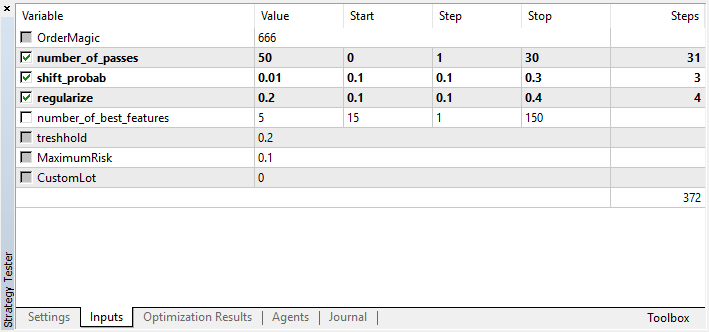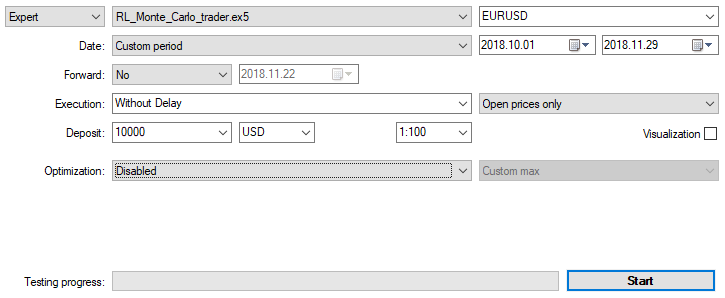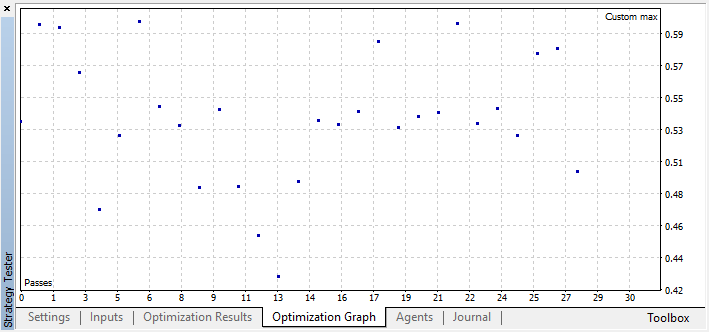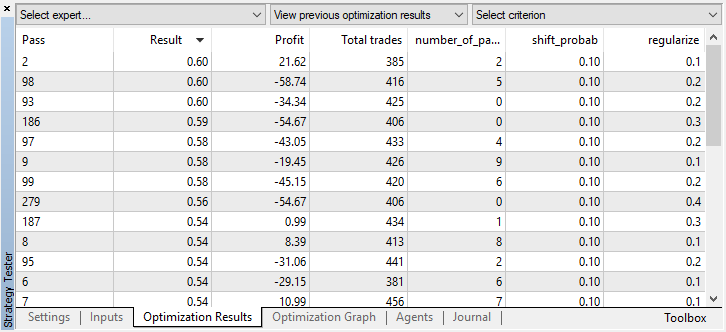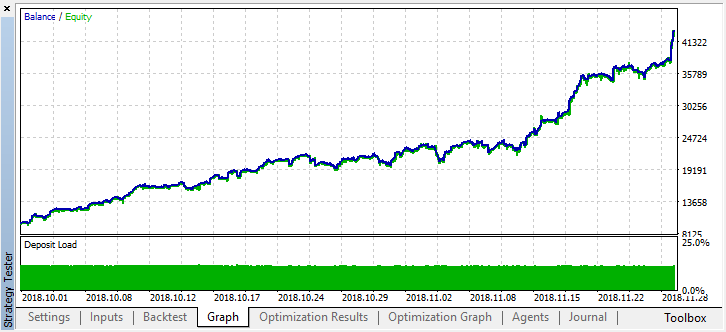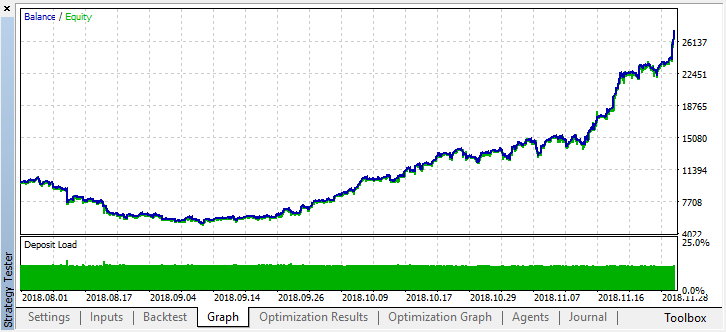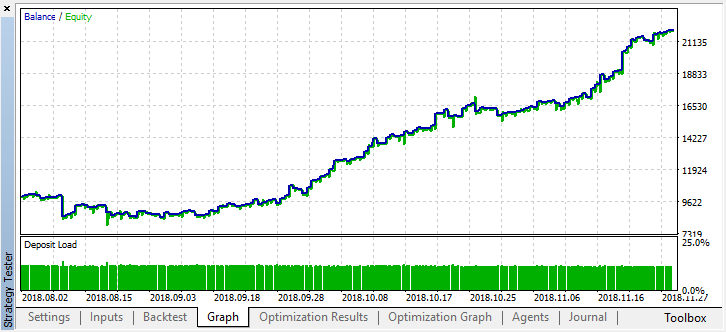`CRLAgents *ag1=new CRLAgents("RlMonteCarloMulti",5,500,number_of_best_features,50,regularize,shift_probab);`

```//+------------------------------------------------------------------+
//| EA 交易初始化函数                                                  |
//+------------------------------------------------------------------+
int OnInit() {
ag1.setAgentSettings(0,500,20,50,regularize,shift_probab);
ag1.setAgentSettings(1,200,15,50,regularize,shift_probab);
ag1.setAgentSettings(2,100,10,50,regularize,shift_probab);
ag1.setAgentSettings(3,50,5,50,regularize,shift_probab);
ag1.setAgentSettings(4,25,2,50,regularize,shift_probab);
return(INIT_SUCCEEDED);
}
```

```//+------------------------------------------------------------------+
//| 计算 Tsignal                                                      |
//+------------------------------------------------------------------+
void calcTsignal() {
Tsignal=0;
for(int i=0;i<ArraySize(ag1.agent);i++) {
CopyClose(_Symbol,0,1,ArraySize(ag1.agent[i].inpVector),ag1.agent[i].inpVector);
ArraySetAsSeries(ag1.agent[i].inpVector,true);
}
}
```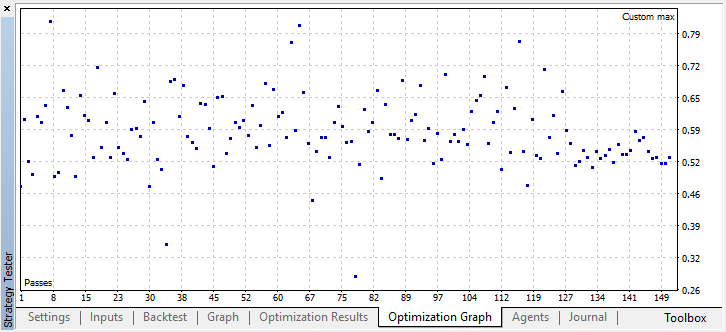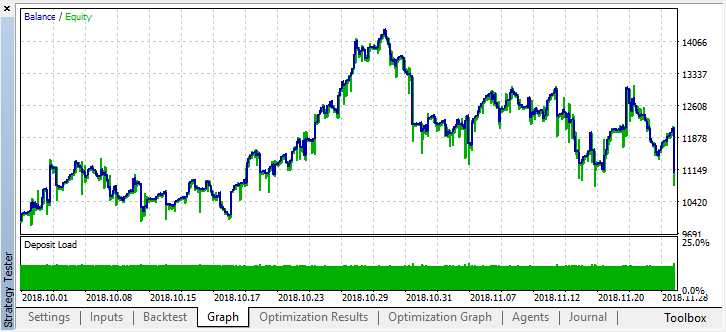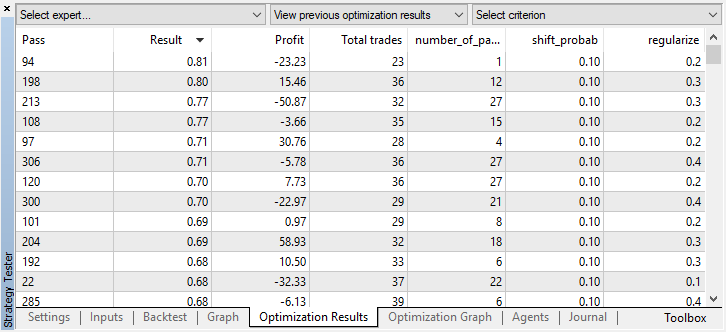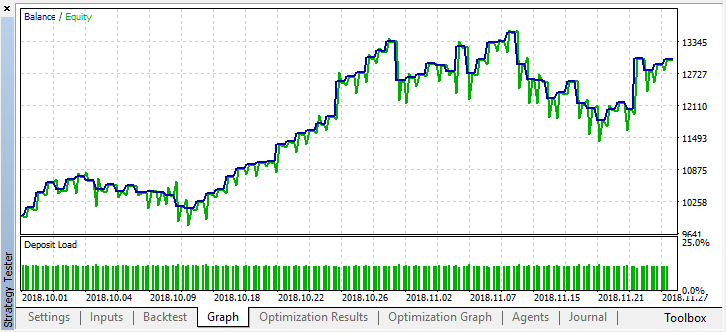```2018.11.30 01:56:40.441 Core 2  2018.11.28 23:59:59   RlMonteCarlo TRAIN LOSS
2018.11.30 01:56:40.441 Core 2  2018.11.28 23:59:59   0.02703 0.20000 0.09091 0.05714 0.14286
2018.11.30 01:56:40.441 Core 2  2018.11.28 23:59:59   RlMonteCarlo OOB LOSS
2018.11.30 01:56:40.441 Core 2  2018.11.28 23:59:59   0.21622 0.23333 0.21212 0.17143 0.19048
```shift-probab设置为0.3，然后在M15上启动优化器，使其在两个月内不带此参数（以查找交易数量平衡）：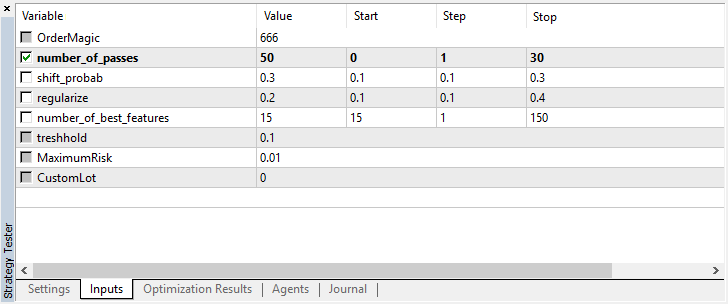```2018.11.30 02:53:17.236 Core 2  2018.11.28 23:59:59   RlMonteCarloMulti TRAIN LOSS
2018.11.30 02:53:17.236 Core 2  2018.11.28 23:59:59   0.13229 0.16667 0.16262 0.14599 0.20937
2018.11.30 02:53:17.236 Core 2  2018.11.28 23:59:59   RlMonteCarloMulti OOB LOSS
2018.11.30 02:53:17.236 Core 2  2018.11.28 23:59:59   0.45377 0.45758 0.44650 0.45693 0.46120
```

测试集上的误差仍然相当大，但是，在0.2的阈值下，该模型在4个月内显示出盈利，尽管它在测试数据上表现出相当不稳定。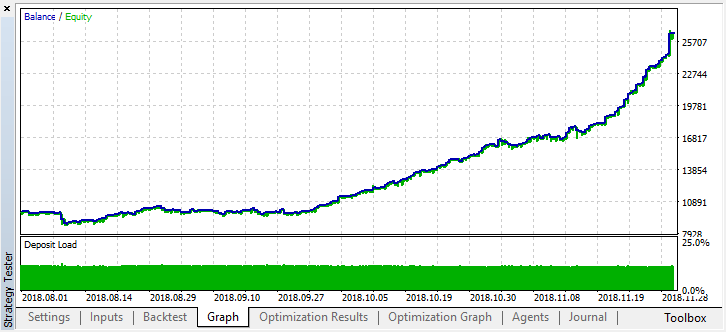## 量化课程

移动端课程